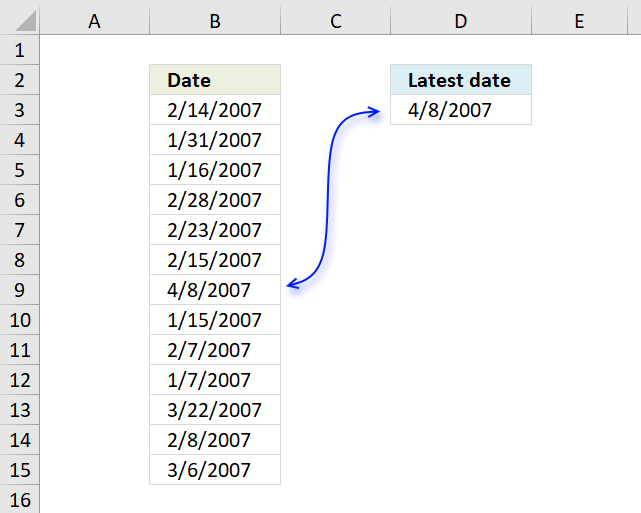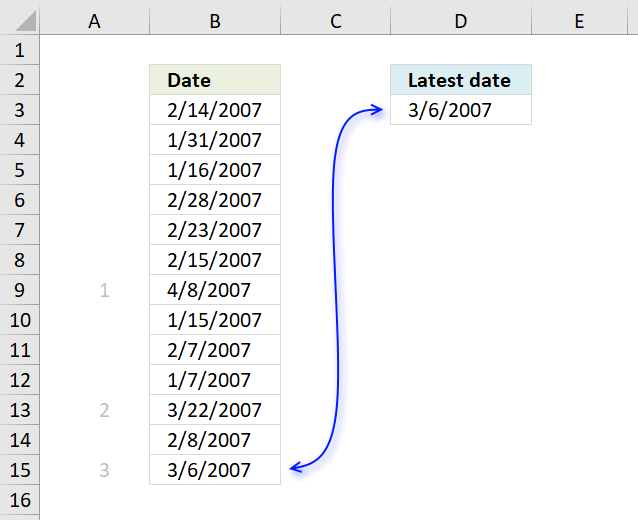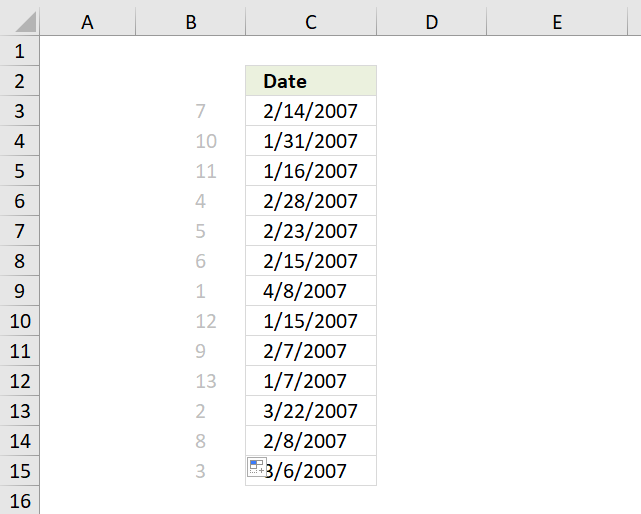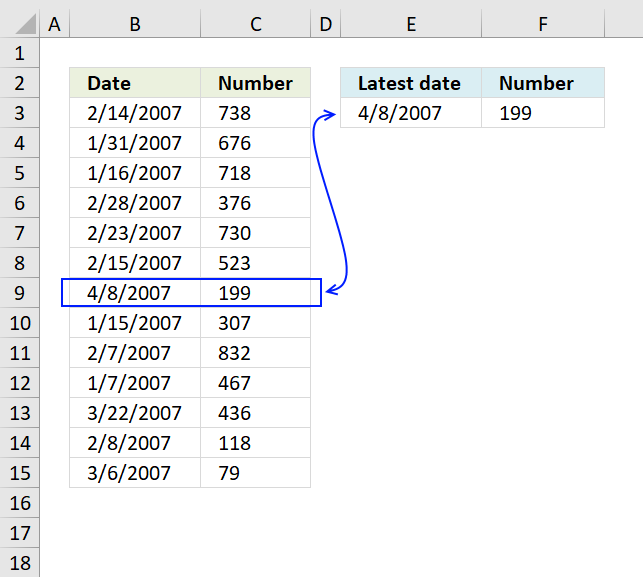Author: Oscar Cronquist Article last updated on January 03, 2023The image above shows a formula in cell D3 that extracts the most recent date in cell range B3:B15.

=MAX(B3:B15)

The MAX function returns the largest number since Excel treats dates as numbers this will work fine. Number 1 is 1/1/1900 and 1/1/2000 is 36526. 1/2/2000 is 36527.

To extract the earliest date use the MIN function:

=MIN(B3:B15)

This returns 1/7/2007 from the list shown above.To get the 3rd latest date use the following formula:

=LARGE(B3:B15, 3)

The LARGE function extracts the k-th largest number in a cell range. k is the second argument in the LARGE function.

To get the second earliest date use the SMALL function:

=SMALL(B3:B15, 2)

1/15/2007 is the second earliest date.If you want to know where specific date is compared to the other dates in a list use the RANK function , in other words, if you were to sort the dates from largest to smallest where in the list would a specific date be located?

For example, 2/14/2007 would be the seventh date if the list shown above were sorted from largest to smallest.

Formula in cell B3:

=RANK(C3,\$C\$3:\$C\$15)

The first argument in the rank function is the value you want to know the rank of. The second argument is the values you want it to be compared with.The image above demonstrates a formula in cell F3 that returns the corresponding value on the same row as the latest date is found.

=INDEX(C3:C15, MATCH(E3,B3:B15,0))

The MATCH function returns the position of the value in cell E3 in cell range B3:B15. It returns 7 because 4/8/2007 is in the seventh position in cell range B3:B15.

INDEX(C3:C15, MATCH(E3,B3:B15,0))

becomes

INDEX(C3:C15, 7)

The INDEX function then returns the value in the seventh position in cell range C3:C15 which is 199.